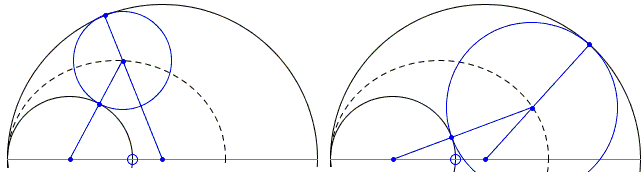# Ellipse in Arbelos

Here is a problem (E 762) from the 1947 American Mathematical Monthly. It was proposed by J. R. Van Andel, Naval Air Experimental Station, Philadelphia, Pa. (The solution below is by Norman Anning, Ann Arbor, Michigan):

 Let A1 and A2 be two circles with radii a1 and a2 and centers (a1, 0) and (a2, 0), respectively, with a2 > a1 > 0. Let C be any circle in the crescent shaped area M between A1 and A2, and tangent to both A1 and A2. The locus of the center of C as it sweeps out M is an ellipse with semiaxes the arithmetic mean (a1 + a2)/2 and the geometric mean √(a1a2) of the radii a1 and a2. If Ct is a circle of radius rt and center Pt(xt, yt) where φt = a1a2 + t² (a2 - a1)², rt = a1a2(a2 - a1) / φt, xt = a1a2(a2 + a1) / φt, yt = 2t rt. then, for any real value of t, Ct lies in M and is tangent to A1, A2, and Ct-1.

### This applet requires Sun's Java VM 2 which your browser may perceive as a popup. Which it is not. If you want to see the applet work, visit Sun's website at https://www.java.com/en/download/index.jsp, download and install Java VM and enjoy the applet.

 What if applet does not run?

Solution

### References

1. J. R. Van Andel,; Norman Anning, American Mathematical Monthly, Vol. 54, No. 9. (Nov., 1947), pp. 547-548.Part (a) is elementary. A glance at a figure shows that the center of C is always in such a position that the sum of its distances from (a1, 0) and (a2 0) is a1 + a2.That the major semiaxis of the ellipse is (a1 + a2)/2 is indeed rather obvious. To determine the minor semiaxis, consider circle C with the center at the topmost point of the ellipse. The triangle of the centers of the three circles will then be isosceles, with the legs equal to (a1 + a2)/2 and the base a2 - a1. The altitude h of the triangle is found from the Pythagorean theorem:

 h² = [(a1 + a2)/2]² - [(a2 - a1)/2]²,

so that indeed, h² = a1a2. (These two facts are easily extended to a more general shape.)

### This applet requires Sun's Java VM 2 which your browser may perceive as a popup. Which it is not. If you want to see the applet work, visit Sun's website at https://www.java.com/en/download/index.jsp, download and install Java VM and enjoy the applet.

 What if applet does not run?

For part (b), let (X, Y) be a generic point on Ct. Then

 (1) (X² + Y²)·φt - 2a1a2(a1 + a2)X - 4t a1a2(a2 - a1)Y + 4(a1a2)² = 0.

Apply to Ct the inversion

 X = 4a1a2 x / (x² + y²), Y = 4a1a2 y / (x² + y²).

Then (1) becomes

 x² + y² - 2(a1 + a2)x - 4t(a2 - a1)y + 4φt = 0,

which may be rewritten as

 (2) (x - a1 - a2)² + (y - 2ta2 + 2ta1)² = (a2 - a1)².

With t as parameter, (2) is the family of equal circles which touch the parallel lines x = 2a1 and x = 2a2. In this family, for every t, the circle Ct is tangent to Ct-1 because the distance between their centers, 2(a2 - a1), is equal to the diameter of either.

Now invert again. Circle C0 inverts into itself and (2) inverts into (1). The line x = 2a2 inverts into the circle A1, the inner boundary of the arbelos. Similarly, x = 2a1 inverts into A2, the outer boundary. Since it is well known that inversion turns circles into circles and preserves contacts, the proof of the stated theorem is complete.

The solution in the Monthly is followed by the following note:

One tracing the history of the problem would find it under arbelos. See R. Johnson's Modern Geometry, for instance. The neatest of the properties, yt = 2trt, appears in book 4 of Pappus's Collection. See Ivor Thomas, Greek Mathematical Works, II (Loeb Classical Library, No. 362), p. 578.

The proposer pointed out that J. Steiner in Geometrische Betrachtungen (1826) discussed, in particular, the chains of circles corresponding to the sequences t = 0, 1, 2, ... and t = 1/2, 3/2, 5/2, ... Williams mentioned several additional properties of the figure which easily follow from the inversion. For instance, the line joining the points of contact of Ct with A1 and A2 passes through the fixed point (2a1a2/(a1 + a2), 0); the common internal tangent of Ct and Ct-1 passes through this same point; the four points consisting of the origin, the centers of A1 and A2, and the above point form a harmonic set. If the diameter of A1 is taken as two-thirds that of A2, then r1 is one-seventh the diameter of A2. Of this particular figure Victor Thébault has stated a very pretty property. Let the diameter of A2 taken along the line of centers of A1 and A2 be OB, and let BM be the tangent from B to the circle A1. Then the circle on BM as diameter is tangent to the circle C1.## Ellipse